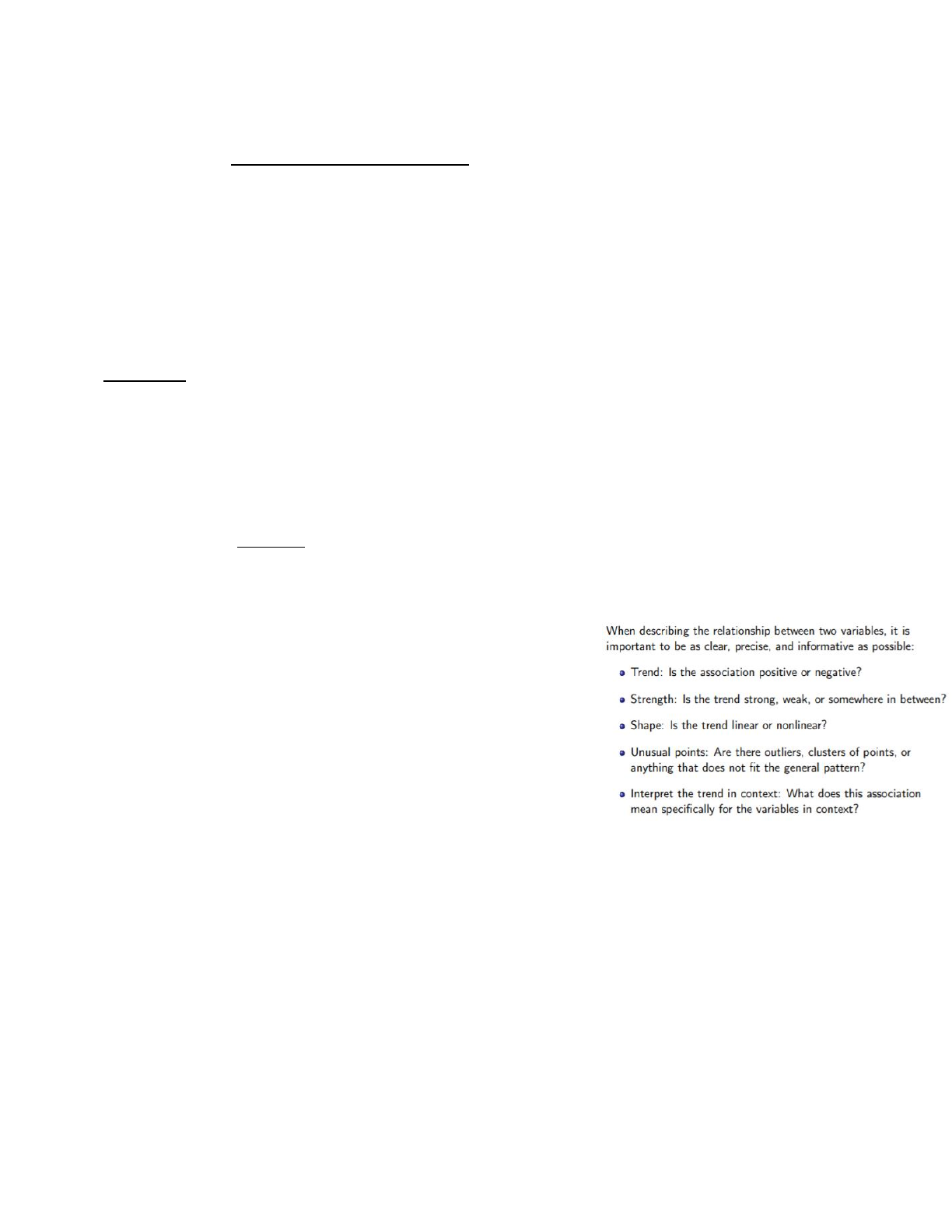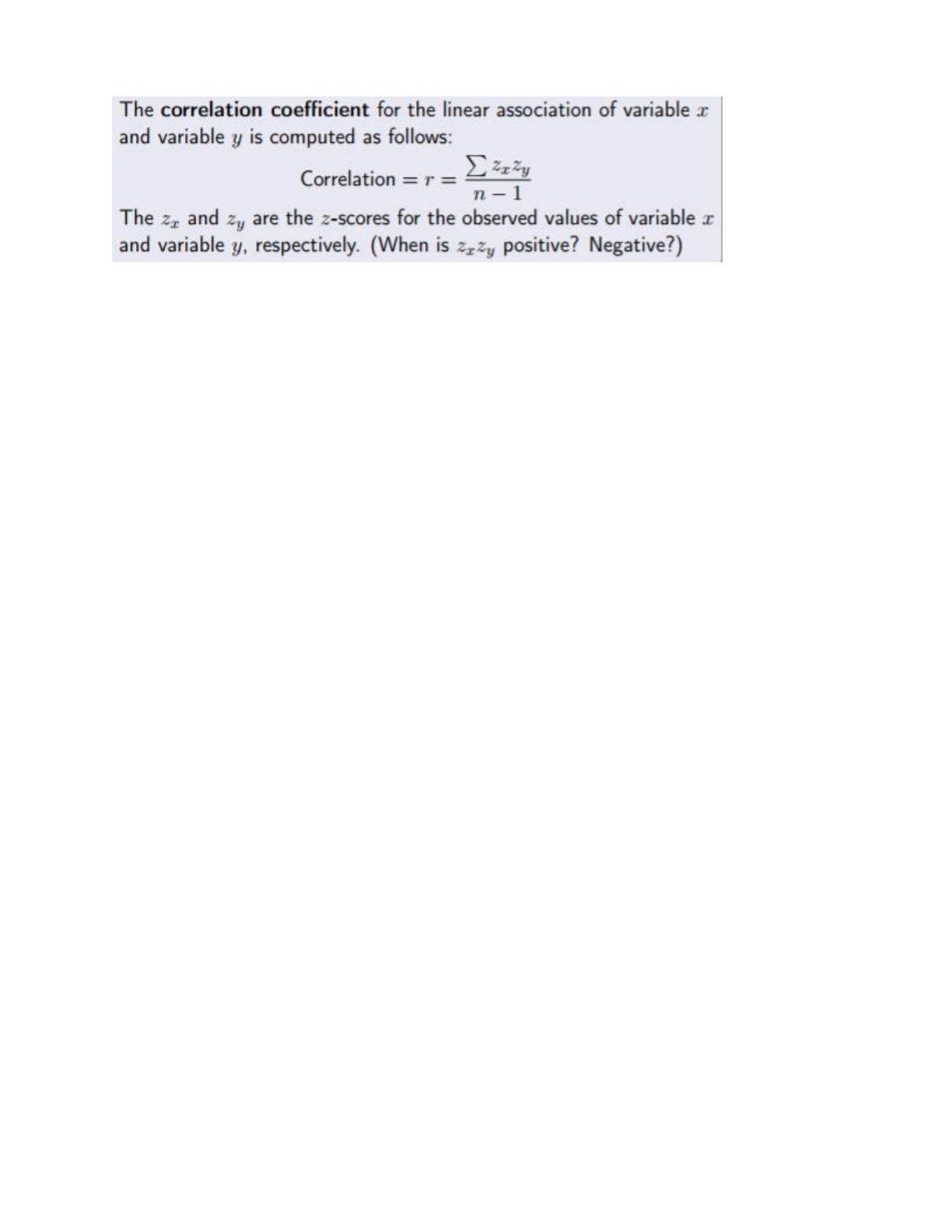Class Notes (1,100,000)
US (480,000)
UCLA (10,000)
STATS (200)
STATS 10 (100)
Lecture 5

# STATS 10 Lecture Notes - Lecture 5: Scatter Plot, Lincoln Near-Earth Asteroid Research, Dependent And Independent Variables

Department
Statistics
Course Code
STATS 10
Professor
Michael Tsiang
Lecture
5

This preview shows page 1. to view the full 5 pages of the document.Chapter 4: Regression Analysis: Exploring Associations Between Variables
Plots to visualize numerical data: dotplots, histograms, stemplots
When describing numerical distribution, consider: shape, center, spread
We will consider questions about the relationship between two numerical variables
Scatterplot: used to plot relationship between two variables; each point represents one observation, and location of point
depends on values of the two variables of interest (one at x-axis, other at y-axis)
Each observation is a PAIR of values
horozontal/vertical axes do not have to be on same scale → just label properly
Bottom left corner does not have to start at (0,0); we want to zoom in on the relationship between the two
variables and not have a lot of empty space
The Big Three (of analyzing relationship between TWO variables via scatterplot)
Trend
Strength
Shape
Trend: association between two variables (associated if there is relationship bewteen them)
Trend of association=general tendency of scatterplot scanning from left to right
Increasing trend (positive association/trend)→ uphil/rising tendency; increases in one variable are
associated w/ increases in the other variable
Decreasing trend (negative association/trend)→ downhill/falling tendency; increases in one variable are
associated with decreases in the other
Describes general tendency, not all individual behavior between two variables
Do not use absolute terms when interpreting trends in context!!!!!
Not always clearly positive or negative!
Strength: of an association (trend)=how closely related two variables are
(amount of scatter in the scatterplot)
Refers to spread of points in the vertical direction
Weak association/trend=large amount of scatter, ie high
vertical variation; trend harder to visually detect
Strong association/trend=little scatter, ie low vertical
variation; trend easier to visually detect
Strength → how well knowing one variable can predict the other
Relative strength (which trend=stronger?) is easier to
distinguish than labelling a trend by itself (subjective!)
Shape: rate of increase/decrease in the trend
Linear: trend always increases/decreases at the same trait; can be
summarized w/ straight line (superimposed
Nonlinear: rate of increase/decrease changes depending on values of variables
E.g. quadratic, exponential, etc; not really covered in course, more focus on linear
Correlation coefficient (correlation): # that measures the strength of the LINEAR association between two numerical
values
Two variables are correlated if they are LINEARLY associated
Only makes sense when trend is LINEAR and both variables are NUMERICAL
Correlation coefficent denoted as r
Always between -1 and 1
Both value and sign are important
Value: strength
If value is close to -1 or +1, association is strong
If value is close to 0, association is weak (correlation=0 if scatterplot shows nonlinear)
Sign: direction
If sign is +, trend is +
If sign is -, trend is -
find more resources at oneclass.com
find more resources at oneclass.com

Unlock to view full version

Only page 1 are available for preview. Some parts have been intentionally blurred.CORRELATION DOES NOT IMPLY CAUSATION! (even if the correlation is close to -1 or +1)
Only a measure of linear association,
Find the mean and standard dev to find z score
Do this for each x and each y
For each observation (scatterplot point), multiply the x and y z-scores
Add all the z-score products up!
Divide this number by n-1 (sample size-1)
Points that add to correlation: values of 2 variables BOTH above or BOTH below means
Points that subtract from correlation: value of one variable above mean and one below mean
Points that do not contribute to correlation have value of at least one variable equal exactly to the
mean
Properties of correlation
The order of variables does not matter
Height vs. hand spain is the same as hand span vs. height
Highlights fact that correlation only tells us about strength of association, cannot imply
causality
Unitless
Only depends on z-scores, units of measurement for each variable don’t affect correlation
inches//pounds is same correlation between cm//kg
Only linear
Correlation does not tell you whether an assocation is linear
Does not tell you shape of graph
If the association is linear, THEN the correlation coefficient is a measure of its strength
If the association is nonlinear, then the correlation coefficient does not have much
interpretability
Statistical modeling
One way to measure a trend
Make an assumption that the trend can be summarized by a math equation
Use observed data to estimate mathematical equation tha tbest describes trend
Analogous to a physical model, except statistical models have inherent uncetainty and must account for
variation
FOR LINEAR TRENDS, assume trend can be summed by a line equation: regression line
Regression line: statistical model that summarizes the linear trend of observed values → also
represents best guess/prediction for any new or future observations
Equation for (straight) line: y=mx+b
m=slope (how steep the line is); change in y for a unit increase in x
b=y-intercept (value of y when x=0)
Statisticians typically write equation of line w/ intercept first: y=a+bx
a=y-intercept
b=slope
Also called least squares line as it’s chosen as to minimize the sum of squared (vertical)
distances of the observed and predicted values → BEST FIT line
find more resources at oneclass.com
find more resources at oneclass.com